## 6.7.21 MultiFFT

### 6.7.21.1 Outline of the node

This node performs Fast Fourier Transforms (FFT) on multichannel speech waveform data.

### 6.7.21.2 Necessary files

No files are required.

### 6.7.21.3 Usage

When to use

This node is used to convert multichannel speech waveform data into spectra and analyze the spectra with time frequency domains. It is often used as preprocessing of feature extractions for speech recognition.

Typical connection

Figure 6.111 shows an example, in which inputs of Matrix<float> and Map<int, ObjectRef> types are provided to the MultiFFT node. The path in Figure 6.111 receives multichannel acoustic signals of Matrix<float> type from the AudioStreamFromWave node. The signals are converted into Matrix<complex<float> > type complex spectra in the MultiFFT node and input to the LocalizeMUSIC node.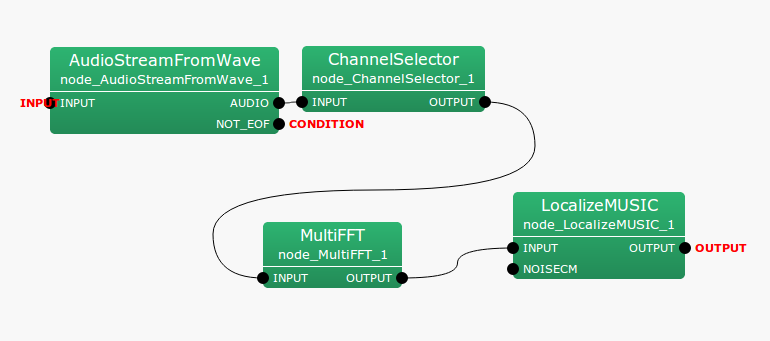Figure 6.111: Example of a connection of MultiFFT

### 6.7.21.4 Input-output and property of the node

Table 6.115: Parameter list of MultiFFT
 Parameter name Type Default value Unit Description LENGTH 512 [pt] Length of signals to be Fourier transformed WINDOW CONJ Type of window function when performing Fourier transform. Select from CONJ, HAMMING, RECTANGLE and HANNING which indicate complex window, hamming window, rectangle window and hanning window respectively. WINDOW_LENGTH 512 [pt] Length of window function when performing Fourier transform.

Input

INPUT

: Matrix<float>  or Map<int, ObjectRef>  types. Multichannel speech waveform data. If the matrix size is $M \times L$, $M$ indicates the number of channels and $L$ indicates the sample numbers of waveforms. $L$ must be equal to the parameter LENGTH.

Output

OUTPUT

: Matrix<complex<float> >  or Map<int, ObjectRef>  types. Multichannel complex spectra corresponding to inputs. When the inputs are Matrix<float>  type, the outputs are Matrix<complex<float> >  type; when the inputs are Map<int, ObjectRef>  type, the outputs are Map<int, ObjectRef>  type. When the input matrix size is $M \times L$, the output matrix size is $M \times L/2 + 1$.

Parameter

LENGTH

: int type. The default value is 512. Designate length of signals to be Fourier transformed. It must be expressed in powers of 2 to meet the properties of the algorithm. Moreover, it must be greater than WINDOW_LENGTH.

WINDOW

: string type. The default value is CONJ. Select from CONJ, HAMMING RECTANGLE and HANNING, which indicate complex, hamming, rectangular and hanning windows, respectively. HAMMING windows are often used for audio signal analyses.

WINDOW_LENGTH

: int type. The default value is 512. Designate the length of a window function. If this value increases, so does the frequency resolution, while the temporal resolution decreases. Intuitively, an increase in window length makes it more sensitive to differences in the pitch of sound while becoming less sensitive to changes in pitch.

### 6.7.21.5 Details of the node

Rough estimates of LENGTH and WINDOW_LENGTH: It is appropriate to analyze audio signals with frame length of $20 \sim 40$ [ms]. If the sampling frequency is $f_{s}$ [Hz] and the temporal length of a window is $x$ [ms], the frame length $L$ [pt] can be expressed as

 $\displaystyle L$ $\displaystyle =$ $\displaystyle \frac{f_{s}x}{1000}$

For example, if the sampling frequency is 16 [kHz], the default value 512 [pt] will correspond to 32 [ms]. Powers of 2 are suited for the parameter LENGTH of Fast Fourier Transform. Select 512. WINDOW_LENGTH, the window function, is set at 400 [pt], corresponding to 25 [ms] when the sampling frequency is 16 [kHz] in some cases to designate a frame length more suited for acoustic analyses.

Shape of each window function: The shape of each window function $w(k)$ is defined by $k$, the index of a sample; $L$, the length of a window function; and $NFFT$, the FFT length. $k$ moves within a range of $0 \leq k < L$. When FFT length is greater than window length, the window function for $NFFT \leq k < L$ is 0.

CONJ, Complex window:

 $\displaystyle w(k)$ $\displaystyle =$ $\displaystyle \left\{ \begin{array}{cr} 0.5 - 0.5 \cos \frac{4kC}{L}, & \mathrm{if}\ \ 0 \leq k < L/4 \\ \sqrt []{\left(1.5 - 0.5 \cos 2C-\frac{4kC}{L}\right) \left(0.5 + 0.5 \cos 2C-\frac{4kC}{L} \right)}, & \mathrm{if}\ \ L/4 \leq k < 2L/4 \\ \sqrt []{\left(1.5 - 0.5 \cos \frac{4kC}{L}-2C\right) \left(0.5 + 0.5 \cos \frac{4kC}{L}-2C \right)}, & \mathrm{if}\ \ 2L/4 \leq k < 3L/4 \\ 0.5 - 0.5 \cos \left( 4C-\frac{4kC}{L} \right), & \mathrm{if}\ \ 3L/4 \leq k < L \\ 0, & \mathrm{if}\ \ NFFT \leq k < L \end{array} \right.,$ $\displaystyle w(k)$ $\displaystyle =$ $\displaystyle \left\{ \begin{array}{cr} 0.5 - 0.5\cos \left( \frac{4k}{L}C \right), & \mathrm{if} 0 \leq k < L/4\\ \sqrt []{1-\left\{ 0.5 - 0.5\cos \left(\frac{2L-4k}{L}C\right) \right\} ^2}, & \mathrm{if} L/4 \leq k < 2L/4\\ \sqrt []{1-\left\{ 0.5 - 0.5\cos \left(\frac{4k-2L}{L}C \right) \right\} ^2}, & \mathrm{if} 2L/4 \leq k < 3L/4\\ 0.5-0.5\cos \left( \frac{4L-4k}{L}C\right), & \mathrm{if} 3L/4 \leq k < L\\ 0, & \mathrm{if} NFFT \leq k < L \end{array} \right.,$

Here, $C = 1.9979$.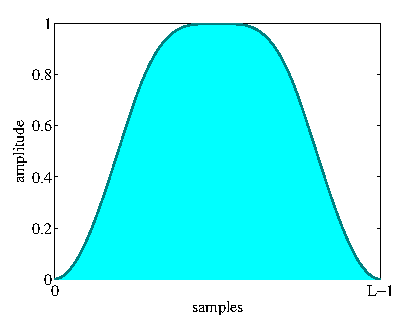Figure 6.112: Shape of complex window function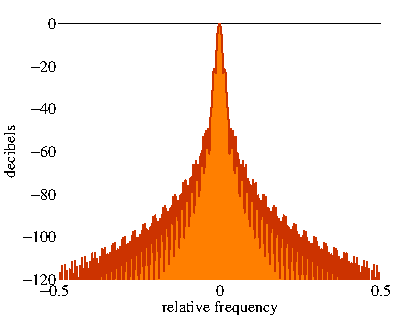Figure 6.113: Frequency response of complex window function

Figures 6.112 and 6.113 show the shape and frequency responses of the complex window function. The horizontal axis in Figure 6.113 indicates the mean relative sampling frequency. Generally, frequency responses of a window function are better if the peak at 0 in the horizontal axis is sharper. Components outside the center of the frequency response indicate the amount of power of other frequency components that leak to a certain frequency bin when performing Fourier transformation. The vertical axis shows the power of other frequencies components that leak into a certain frequency bin when performing Fourier transformation.

HAMMING, Hamming window:

 $\displaystyle w(k)$ $\displaystyle =$ $\displaystyle \left\{ \begin{array}{cr} 0.54 - 0.46 \cos \frac{2 \pi k}{L-1}, & \mathrm{if}\ \ 0 \leq k < L,\\ 0, & \mathrm{if}\ \ L \leq k < NFFT \end{array} \right.$

Here, $\pi$ indicates a circular constant.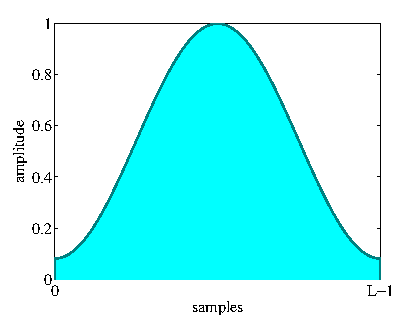Figure 6.114: Shape of hamming window function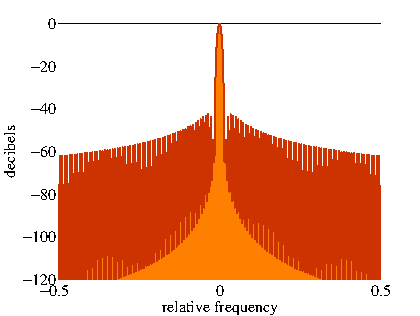Figure 6.115: Frequency response of hamming window function

Figures 6.114 and 6.114 show the shape and frequency responses of the hamming window function, respectively.

RECTANGLE, Rectangle window:

 $\displaystyle w(k)$ $\displaystyle =$ $\displaystyle \left\{ \begin{array}{cr} 1, & \mathrm{if}\ \ 0 \leq k < L\\ 0, & \mathrm{if}\ \ L \leq k < NFFT \end{array} \right.$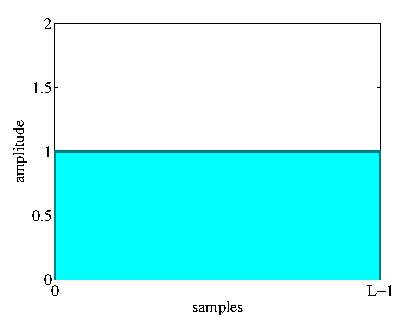Figure 6.116: Shape of rectangle window function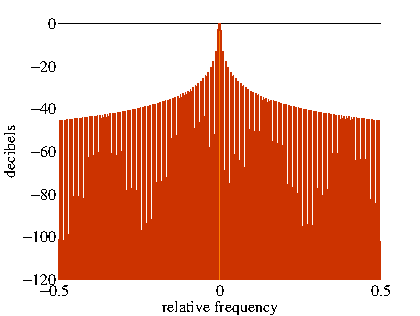Figure 6.117: Frequency response of rectangle window function

Figures 6.116 and 6.116 show the shape and frequency responses of the rectangular window function, respectively.

HANNING, hanning window:

 $\displaystyle w(k)$ $\displaystyle =$ $\displaystyle \left\{ \begin{array}{cr} 0.5 - 0.5 \cos \frac{2 \pi k}{L-1}, & \mathrm{if}\ \ 0 \leq k < L, \\ 0, & \mathrm{if}\ \ L \leq k < NFFT \end{array} \right.$